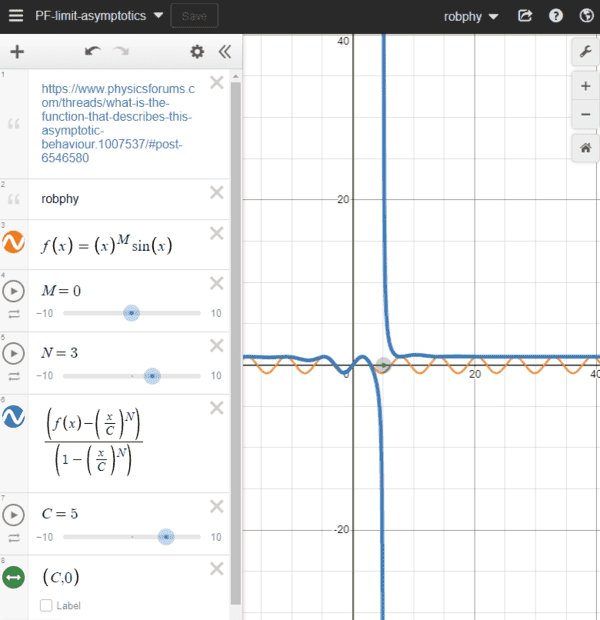# What is the function that describes this Asymptotic behaviour?

• I
Gold Member
I would like to find a function such that for

$$a(x) \rightarrow 1~\text{for}~(x \gg x_c)$$

$$a(x) \rightarrow f(x)~\text{for}~(x \ll x_c)$$

What could be the ##a(x)## ? I have tried some simple functions but could not figure it out. Maybe I am just blind to see the correct result.

Mentor
2022 Award
I would like to find a function such that for

$$a(x) \rightarrow 1~\text{for}~(x \gg x_c)$$

$$a(x) \rightarrow f(x)~\text{for}~(x \ll x_c)$$

What could be the ##a(x)## ? I have tried some simple functions but could not figure it out. Maybe I am just blind to see the correct result.
This is far too vague to give a reasonable answer. How about
$$a(x):=\begin{cases} f(x)&\text{ if }x\leq x_c\\1&\text{ if }x> x_c \end{cases}$$

Gold Member
This is far too vague to give a reasonable answer.
How so ?
Hmm..that also works I guess

Gold Member
What if I say, finding simplest possible $a(x)$. Is that makes sense ? Of course there could be infinetly many functions, but I am looking for least complicated/simplest one

Mentor
What if I say, finding simplest possible $a(x)$. Is that makes sense ? Of course there could be infinetly many functions, but I am looking for least complicated/simplest one
The one that @fresh_42 suggested is pretty simple. Without knowing what f(x) is, you probably won't find anything simpler than that.

•pbuk
Gold Member
No, I am looking for one single function, I mean. When I take the asymptotic behavior, it produces the results I have mentioned.

I am not looking for the same answer in a different form.

Homework Helper
Gold Member
Do you want it to be a continuous function of x?
Consider this:
calculate ##r = (\arctan(x)/\pi + 0.5)##
calculate ##h(x) = f(x)(1-r) + r##

Last edited:
Gold Member
Okay, I have found. It seems the answer is

For $$a(x) = \frac{f(x)x_c - x}{x_c - x}$$
$$a(x) = 1~\text{for}~x \gg x_c$$
$$a(x) = f(x)~\text{for}~x \ll x_c$$

Try ##a(x)=\frac{2.arctan(x)}{\pi}##

Mentor
2022 Award
Okay, I have found. It seems the answer is

For $$a(x) = \frac{f(x)x_c - x}{x_c - x}$$
$$a(x) = 1~\text{for}~x \gg x_c$$
$$a(x) = f(x)~\text{for}~x \ll x_c$$
Still heavily depends on ##f(x)##. And what do you mean by ##x\ll x_c##? What if ##x\to -\infty ##?

This doesn't solve your problem unless you make several assumptions on ##f(x)## which you didn't tell us about!

Gold Member
Well, currently we don't know the ##f(x)## but we know that it does not contain ##x_c##. BTW ##x_c## is just a constant, and ##x## ranges from ##[0, \infty)##. I am not interested the behaviour of the ##f(x)## as ##x \ll x_c## I am just interested in the behaviour of the ##a(x)## as a whole. ##f(x)## could also be a some constant. Or linearly dependent on ##x## but its not much complicated...

I guess you mean that if we open up the ##f(x)##, the behavior of the asymptotes will change?

Mentor
2022 Award
I guess you mean that if we open up the ##f(x)##, the behavior of the asymptotes will change?

Yes. I haven't checked in detail all possibilities, but it seemed you assumed that ##f(x)## is bounded in some sense and continuous. Otherwise, it could "overwrite" the rest of the formula.

Gold Member
Yes. I haven't checked in detail all possibilities, but it seemed you assumed that ##f(x)## is bounded in some sense and continuous. Otherwise, it could "overwrite" the rest of the formula.
Well yes its. Also it seems that the f(x) must be in the 0th order or 1st order. Otherwise the asymptotic relation fails. But of course this is true for this function. I am not sure we can find a more general one

Homework Helper
Gold Member
Okay, I have found. It seems the answer is

For $$a(x) = \frac{f(x)x_c - x}{x_c - x}$$
$$a(x) = 1~\text{for}~x \gg x_c$$
$$a(x) = f(x)~\text{for}~x \ll x_c$$
You might like to play around with
https://www.desmos.com/calculator/da9wzphuxc

[You can rescale the axes by shift-drag near each axis.]